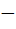Question 111

In a triangle ABC, a= 4, b =3, angle A = 60° then c is a root of the equation

a) c23c7 = 0

b) c2 + 3c + 7 = 0

c) c23c + 7 = 0

d) c2 + 3c7 = 0

AIEEE 2002